Complex-Number Representation of Polarization States

As we've seen from the article about polarization states, a light beam can be represented by its electric field vector E(r,t), which can be written:

E = A cos(ωt - kr)

Being a transverse wave, the electric field vector must lie in the xy plane. So it can be decomposed into two mutually independent orthogonal components Ex and Ey:

Ex = Ax cos(ωt - kz + δx)
Ey = Ay cos(ωt - kz + δy)

where we have used two independent and positive amplitudes Ax and Ay, and have added two independent initial phases δx and δy.

Since the x component and the y component can oscillate independently at the same frequency ω, we must consider the effect produced by the vector addition of these two oscillating orthogonal components.

Without loss of generality, we consider the time evolution of the electric field vector at the origin z = 0. Thus the two components are written

Ex = Ax cos(ωt + δx)
Ey = Ay cos(ωt + δy)

For mathematical simplicity, the monochromatic plane wave as described by the two equations above can be written in complex exponential form: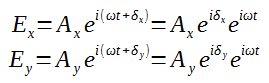We can see that the polarization state of a light beam can be described in terms of the amplitudes and the phase angles of the x and y components.

And we define a complex number χ asWe define a relative phase δ (-π < δ ≤ π) as

δ = δy - δx

So χ can be written as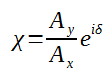Here χ is based on polar coordinate system and it contains all the information about the polarization state of the wave. As shown in the figure below.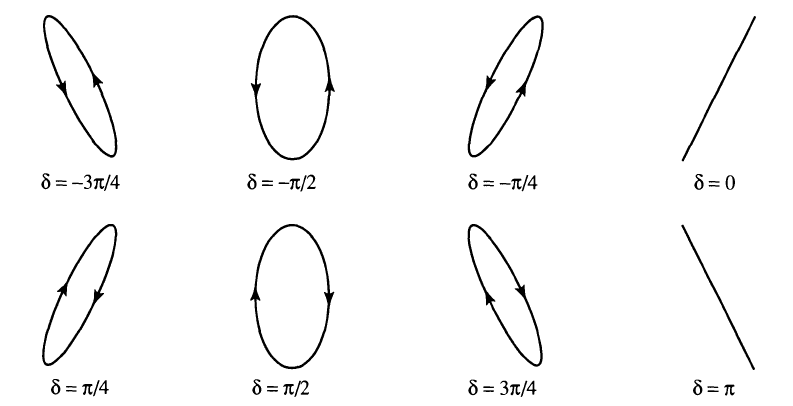Based on Euler's formulaWe can convert χ from the exponential complex number form into x-y orthogonal coordinate system: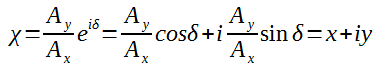And we get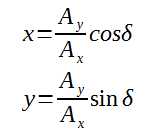We can then plot χ on the x-y complex plane, and its corresponding polarization state:Each point on the complex plane represents a unique state of polarization.

1. The origin point (0,0)

This means

x = Ay/Ax cosδ = 0
y = Ay/Ax sinδ = 0

this means that Ay = 0. So this is a linearly polarized light along the x direction.

2. Points on the horizontal x axis (except (0,0))

This means

x = Ay/Ax cosδ = A (A is a real number)
y = Ay/Ax sinδ = 0

Since y = 0 and x ≠ 0, so sinδ = 0 (δ = 0 (x>0) or π (x<0)), which means a linearly polarized light.

3. Points on the vertical y axis (except (0,0))

This means

x = Ay/Ax cosδ = 0
y = Ay/Ax sinδ = A (A is a real number)

Since x = 0 and y ≠ 0, so cosδ = 0 (δ = π/2 or -π/2).

For points (0,1) and (0,-1), Ay = Ax, and it is a left-handed circular polarization and right-handed circular polarization respectively.

4. Points not on either axis

In this case, the polarization state is an ellipse and has different degree of ellipticity depending on the value of Ay/Ax.

If y > 0, then it is left-handed, and if y < 0, then it is right handed.